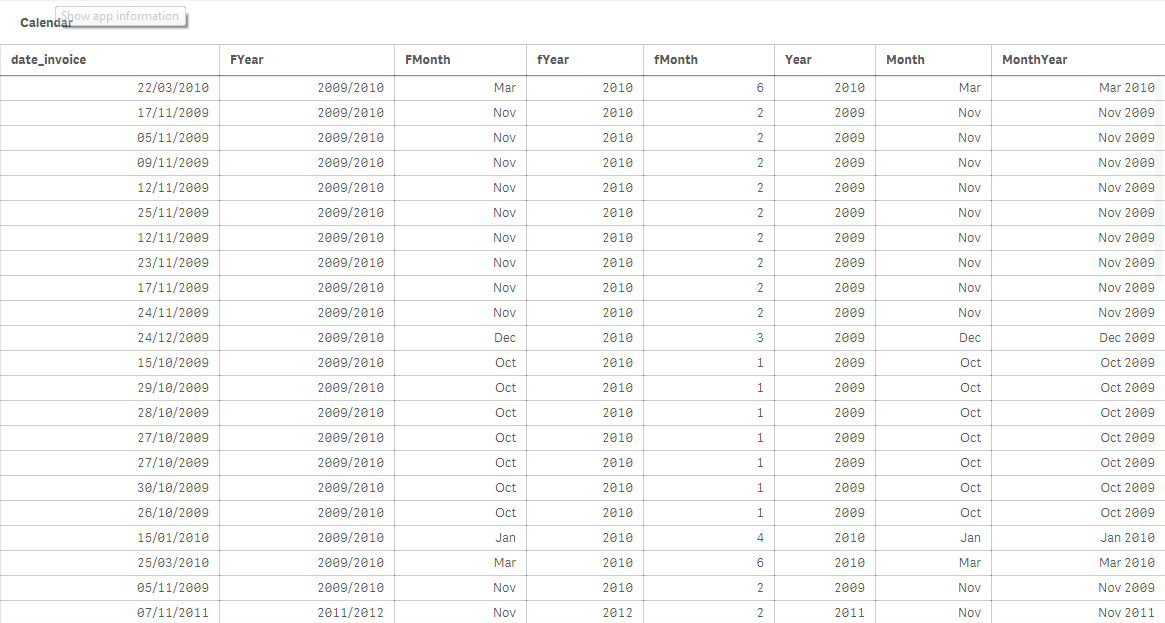# New to Qlik Sense

Discussion board where members can get started with Qlik Sense.

Announcements
BARC’s The BI Survey 19 makes it official. BI users love Qlik. GET REPORT
HighlightedNot applicable

## Using Rangesum for YTD calc

Hi Experts,

Could someone help me debug this expression:

I have created a master calendar and using that to calculate YTD.

Thanks

Swetha

Tags (2)
7 RepliesMVP & Luminary

## Re: Using Rangesum for YTD calc

 ,-(fMonth-1)

 fmonth-1

I assume these two are supposed to be references to the same field or variable. Make sure to use the exact case sensitive name. fMonth and fmonth are two different names.

Oh, and you can get rid of the AddMonths function. You're already using MonthStart and that accepts an offset parameter that works the same as the second parameter to AddMonths.

talk is cheap, supply exceeds demandNot applicable

## Re: Using Rangesum for YTD calc

Hi Gysbert,

Thanks for the response. My pivot table looks like this :The user selects the month and year for which they want to see the year to date calculation. For the YTD calc this is the expression -

Sum({\$<[number]={'*SINV*'},[res_partner.name]={*}-{''}, [date_invoice]={'>=\$(=MonthStart(max(MonthYear),-(fMonth-1)))<\$(=MonthEnd(max(MonthYear)))'}>}amount_untaxed)-

Sum({\$<[number]={'*SREF*'},[res_partner.name]={*}-{''}, [date_invoice]={'>=\$(=MonthStart(max(MonthYear),-(fMonth-1)))<\$(=MonthEnd(max(MonthYear)))'}>}amount_untaxed)

The dimenion is Currency. Could you please let me know if i am working in the right direction to derive the YTD. Is there anything that i am missing here because this expression just refuses to give me the correct values rather it just doesn't give me a  cumulative value.

Thanks

SwethaMVP & Luminary

## Re: Using Rangesum for YTD calc

That looks like a MTD expression to me. I'd expect YearStart to calculate a YTD:

Sum({\$<[number]={'*SINV*'},[res_partner.name]={*}-{''}, [date_invoice]={'>=\$(=YearStart(max(MonthYear),0,-(fMonth-1)))<\$(=MonthEnd(max(MonthYear)))'}>}amount_untaxed)-

Sum({\$<[number]={'*SREF*'},[res_partner.name]={*}-{''}, [date_invoice]={'>=\$(=YearStart(max(MonthYear),0,-(fMonth-1)))<\$(=MonthEnd(max(MonthYear)))'}>}amount_untaxed)

And I don't understand what fMonth is. Can you post a small qlik sense document that makes clear with with kind of data and dates your working?

talk is cheap, supply exceeds demandNot applicable

## Re: Using Rangesum for YTD calc

The fiscal year starts from Oct hence Jan 2016 YTD calculation should be Oct 2015 + Nov 2015 + Dec 2015 + Jan 2016. Hence i have a master calendar created for the same which contains dates as shown in the image:For every invoice date there is a fMonth calculated which indicates how many months should be back tracked for YTD calculation. That is for example Jan 2016 the fMonth will be 4.

Thanks

SwethaMVP & Luminary

## Re: Using Rangesum for YTD calc

These apply to Qlik Sense as well.

talk is cheap, supply exceeds demandNot applicable

## Re: Using Rangesum for YTD calc

I have tried the Range Sum Aggr combination, but that works when there is only one dimension. i have two dimensions (month and currency).

Thanks

SwethaMVP & Luminary

## Re: Using Rangesum for YTD calc

Then try the asof table

talk is cheap, supply exceeds demand